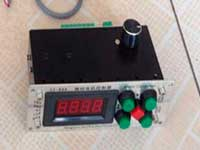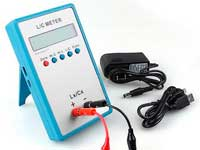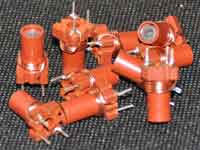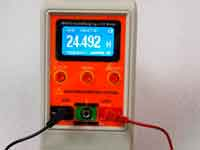Rating 0.00 (0 Votes)

# Features of calculation of power supply chokesVery often, beginner radio amateur has a need for calculating the choke on a ferrite toroid core for switching mode power supply or another circuit in which high currents circulate. In this case, by requesting a "calculation of inductance on a ferrite ring", it is very likely that he will be redirected to our online calculator. Using this calculator or the Coil32 app to calculate the inductance of the choke, ham radio often gets the results didn't match with the handbooks nor didn't match with the practical results. At the same time, it may even result in the burnout of transistors and curses against the Coil32's developers. What's the matter? Let's see...

The reason lies in the fact that beginner amateurs often either do not know or have a simplified view of the features of magnetization of the ferrite. So, take the ferrite core, insert it into the air core coil and its inductance increases by a value of the relative magnetic permeability of the core. Right? True, but not quite! The fact that to describe the properties of ferrite there are several magnetic permeabilities, said that is not so simple. The magnetic properties of the ferrite are most fully described by a family of so-called magnetization BH curves, otherwise called "hysteresis loop".What is the process of magnetization/demagnetization of ferrite, what is the remanence flux density Br, the coercive magnetic field strength Hc, the saturation flux density, major hysteresis loop , etc have already sufficiently described and you can read about it following the references below. We will focus here on how the magnetic permeability of the core is changing during its magnetization since this parameter is used for calculations in the Coil32 app. Here is a partial list of concepts magnetic permeability:•  Relative and absolute magnetic permeability. In fact, the only difference is the multiplier µ0 = 4π*10-7 which really co-ordinates in the SI system the electromagnetic units and the length unit and is conventionally referred to as the magnetic permeability of vacuum or magnetic constant.
• Apparent or normal magnetic permeability is the B/H ratio at the some point on the magnetization curve and at the static magnetic field.
• In general case, the value of relative magnetic permeability is proportional to the slope of the tangent to the magnetization curve or derivative of the BH curve at the given point. This value is called the differential magnetic permeability or incremental permeability. It is not constant and changes dynamically with the movement on the magnetization curve.
• Initial magnetic permeability µi is characterized by the slope of the initial magnetization curve  at the origin. Usually, this value is given in the manufacturer's data.
• The maximum magnetic permeability µmax. When the magnetization of the ferrite increases, its magnetic permeability increases, reaching some maximum and then begins to decrease. The value of the maximum magnetic permeability is usually significantly more than initial. You can also find it in the data-sheets of the ferrite cores.
• Dynamic magnetic permeability is the average incremental permeability. Describes how the coil inductive reactance will be increased if the air around it will be replaced by ferrite. I.e. that is what we are interested in. If the ferrite is placed in a relatively low alternating magnetic field, not driving it into the major hysteresis loop, the magnetization BH curve (minor hysteresis loop) can be approximately represented as an ellipse. Then, with sufficient approximation, we can assume that the dynamic magnetic permeability is characterized by the inclination of the major axis of this ellipse.
• Effective magnetic permeability µeff. This value applies not to the ferrite and to the core with an open magnetic circuit.

For low fields, without DC bias, ferrite magnetization curve is usually as a curve  and in this case the value of the dynamic magnetic permeability close to the value of the initial magnetic permeability of the ferrite. Therefore, in low-current circuits with a relatively small error in the calculations, you can use the value of the initial magnetic permeability, how program Coil32 does.

Another case is the inductor in the switching power supply. The commonly used ferrites have a relatively low value of saturation flux density (about 0.3 T), so in the power switching circuit the inductor is switched between the maximum value of the field when it almost gets a saturation and zero-field value, when it is demagnetized to a value of residual induction (curve ). As we can see the slope of the major axis of the ellipse 4 is much smaller than that of the ellipse 3. In other words, the magnetic permeability of the core in this mode is greatly reduced. The situation becomes worse if the choke core has DC bias (curve ). The major hysteresis loop of the real ferrite is more rectangular than on our schematic image and, in the end, the dynamic magnetic permeability of the power inductor on a ferrite ring falls to several units. As if there is no the ferrite! In the end, the inductive reactance of the inductor decreases, the current increases dramatically (which results in an even larger decrease in µ!), the key transistor heats up and burnout. The calculations of Coil32 for this choke give an absolutely wrong result. Because, we used to calculate the value of initial magnetic permeability, and in a real circuit, the permeability is two to three orders smaller. You will get the same situation if you will measure the relative permeability of the toroid by the trial winding.

The solution is to use a ferrite core with the interrupted magnetic circuit. In the case of the ferrite ring, it is necessary to break it in half and then glue that two half with the non-magnetic gap. The major hysteresis loop of such a core becomes more sloping , the residual induction is much less [B'r], the effective magnetic permeability is also less than that of the core without a gap. However, the curve of magnetization  shows that the dynamic magnetic permeability of this inductor is much higher than that of similar, but with a core without a gap. It has permeability about 50..100. Coil32 also is unable to calculate this choke, since it does not take into account the non-magnetic gap. Another solution is the use of special rings for the power supply as powdered iron toroids (not ferrite). Such toroids can be found in pulse power supply units and motherboards of computers. The non-magnetic "gap" in such ring is distributed along its length.

Conclusion. The Coil32 program calculates only low-current ferrite toroid coil working in low magnetic fields. For the power chokes calculation, it is necessary to use a completely different methodology.

References:

Сomments from anonymous guests are enabled with moderation.

#### Coil Winding Machine###### US \$187.53
The system consists of individual components including a controller, a stepping motor, a foot switch, power adapter and output shaft. This design makes it very easy for you to integrate the system into your own project.

#### LC Meter LC200A###### US \$37.99
Measuring Capacitance Range:
0.01 pF - 100mF
Measuring Inductance Range:
0.001 uH - 100H
Measurement accuracy:1%.

#### RF Inductors###### US \$6.43
Variable Ferrite Core Inductor coil set 3.5Turns 25-100MHZ

#### Auto Ranging LCR Meter###### US \$63.07
Meter can measure 0.00pF to 100.00mF capacitance, 0.000uH to 100.00H inductance and 0.00? to 10.000M? resistance. Very suitable for small value capacitor and inductor measurement.